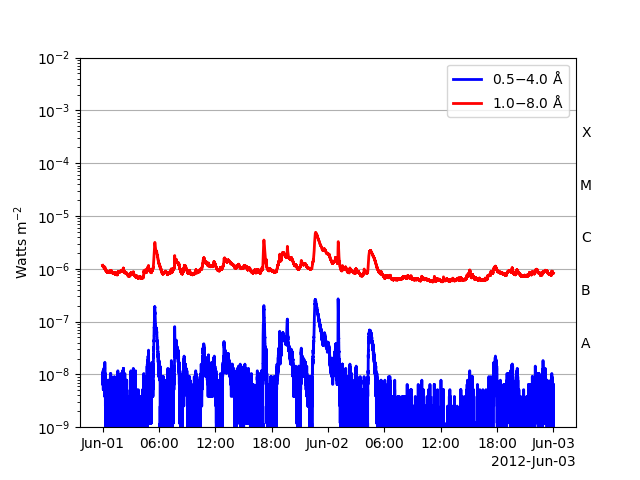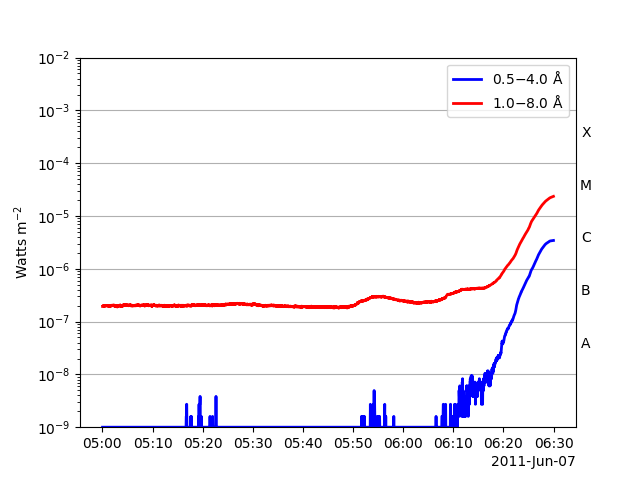Interacting with Data Using SunPy TimeSeries¶

This is an early run-through of the basic functionality of the SunPy TimeSeries class. This is intended primarily to demonstrate the current interface for discussion of the final implementation. Much of the code will be changes as the class is developed.

```import datetime
import copy
from collections import OrderedDict

import numpy as np
from pandas import DataFrame
import matplotlib.pyplot as plt

import astropy.units as u
from astropy.time import Time, TimeDelta
from astropy.table import Table

import sunpy.data.sample
import sunpy.timeseries
from sunpy.time import TimeRange, parse_time
from sunpy.net import Fido, attrs as a
```

Creating a TimeSeries from a file can be done using the factory.

```ts_eve = sunpy.timeseries.TimeSeries(sunpy.data.sample.EVE_TIMESERIES, source='EVE')
ts_goes = sunpy.timeseries.TimeSeries(sunpy.data.sample.GOES_XRS_TIMESERIES, source='XRS')
ts_lyra = sunpy.timeseries.TimeSeries(sunpy.data.sample.LYRA_LEVEL3_TIMESERIES, source='LYRA')
ts_noaa_ind = sunpy.timeseries.TimeSeries(
sunpy.data.sample.NOAAINDICES_TIMESERIES, source='NOAAIndices')
ts_noaa_pre = sunpy.timeseries.TimeSeries(
sunpy.data.sample.NOAAPREDICT_TIMESERIES, source='NOAAPredictIndices')
ts_norh = sunpy.timeseries.TimeSeries(sunpy.data.sample.NORH_TIMESERIES, source='NoRH')
ts_rhessi = sunpy.timeseries.TimeSeries(sunpy.data.sample.RHESSI_TIMESERIES, source='RHESSI')
ts_gbm = sunpy.timeseries.TimeSeries(sunpy.data.sample.GBM_TIMESERIES, source='GBMSummary')
# Note: for some FITS files a source can be determined implicitly, however it
# is good practice to delcare it explicitly when possible.
```

You can create a list of TimeSeries objects by using multiple files. First however, we shall download these files using `Fido`.

```goes = Fido.search(a.Time("2012/06/01", "2012/06/04"), a.Instrument("XRS"))
goes_files = Fido.fetch(goes)

# Using these new files you get a list:
lis_goes_ts = sunpy.timeseries.TimeSeries(goes_files[:2], source='XRS')
lis_goes_ts = sunpy.timeseries.TimeSeries(goes_files, source='XRS')
# Using concatenate=True kwarg you can merge the files into one TimeSeries:
combined_goes_ts = sunpy.timeseries.TimeSeries(goes_files, source='XRS', concatenate=True)
fig, ax = plt.subplots()
combined_goes_ts.plot()
# Note: ATM we only accept TimeSeries of a single class being created together
# with the factory. The issue is that several source filetypes don't contain
# metadata that enables us to reliably implicitly gather the source and ATM the
# source is given as a single keyword argument for simplicity. But you can merge
# different TimeSeries classes using concatenate.
# Debate: are we OK for one source at a time?
```You can concatenate manually:

```combined_goes_ts = lis_goes_ts.concatenate(lis_goes_ts)
fig, ax = plt.subplots()
combined_goes_ts.plot()
```The TimeSeries object has 3 primary data storage components: data (pandas.DataFrame): stores the data. meta (OrderedDict): stores the metadata (like the Map) units (OrderedDict): stores the units for each column, with keys that match the name of each column. These can be accessed like on the map:

```ts_lyra.data
ts_lyra.meta
ts_lyra.units
# There are a couple of other useful properties you can quickly get:
ts_lyra.time_range
ts_lyra.index
ts_lyra.columns
# Further data is available from within the metadata, you can filter out for a
# key using the TimeSeriesMetaData.get() method:
combined_goes_ts.meta.get('telescop')
# Note: this returns a TimeSeriesMetaData object, to get a list of just the
# values for this key use the values property of the metadata:
combined_goes_ts.meta.get('telescop').values()
# Note: this always returns a list because there may be one or more results.
```

The ID used in the data Pandas DataFrame object will be a datetime, as can be seen using ts_lyra.index. You can access a specific value within the TimeSeries data DataFrame using all the normal Pandas methods. For example, the row with the index of 2015-01-01 00:02:00.008000:

```ts_lyra.data.loc[parse_time('2011-06-07 00:02:00.010').datetime]
# Pandas will actually parse a string to a datetime automatically if it can:
ts_lyra.data.loc['2011-06-07 00:02:00.010']
# Pandas includes methods to find the indexes of the max/min values in a dataframe:
lyra_ch1_max_index = ts_lyra.data['CHANNEL1'].idxmax()
lyra_ch1_min_index = ts_lyra.data['CHANNEL1'].idxmin()
```

```combined_goes_ts.meta
print(combined_goes_ts.meta)
print(combined_goes_ts.meta.to_string(2))
```

Out:

```|-------------------------------------------------------------------------------------------------|
|TimeRange                  | Columns         | Meta                                              |
|-------------------------------------------------------------------------------------------------|
|2012-06-01 23:59:59.398999 | xrsa            | simple: True                                      |
|            to             | xrsb            | bitpix: 8                                         |
|2012-06-02 23:59:57.661999 |                 | naxis: 0                                          |
|                           |                 | extend: True                                      |
|                           |                 | date: 26/06/2012                                  |
|                           |                 | numext: 3                                         |
|                           |                 | telescop: GOES 15                                 |
|                           |                 | instrume: X-ray Detector                          |
|                           |                 | object: Sun                                       |
|                           |                 | origin: SDAC/GSFC                                 |
|                           |                 | ...                                               |
|-------------------------------------------------------------------------------------------------|
|2012-06-02 23:59:59.708999 | xrsa            | simple: True                                      |
|            to             | xrsb            | bitpix: 8                                         |
|2012-06-03 23:59:55.928999 |                 | naxis: 0                                          |
|                           |                 | extend: True                                      |
|                           |                 | date: 26/06/2012                                  |
|                           |                 | numext: 3                                         |
|                           |                 | telescop: GOES 15                                 |
|                           |                 | instrume: X-ray Detector                          |
|                           |                 | object: Sun                                       |
|                           |                 | origin: SDAC/GSFC                                 |
|                           |                 | ...                                               |
|-------------------------------------------------------------------------------------------------|

|-------------------------------------------------------------------------------------------------|
|TimeRange                  | Columns         | Meta                                              |
|-------------------------------------------------------------------------------------------------|
|2012-06-01 23:59:59.398999 | xrsa            | simple: True                                      |
|2012-06-02 23:59:57.661999 | xrsb            | bitpix: 8                                         |
|                           |                 | ...                                               |
|-------------------------------------------------------------------------------------------------|
|2012-06-02 23:59:59.708999 | xrsa            | simple: True                                      |
|2012-06-03 23:59:55.928999 | xrsb            | bitpix: 8                                         |
|                           |                 | ...                                               |
|-------------------------------------------------------------------------------------------------|
```

The TimeSeries objects can be visualised using peek():

```fig, ax = plt.subplots()
ts_goes.plot()
# And you can use subplots:
ts_eve.peek(subplots=True)
```
••An individual column can be extracted from a TimeSeries:

```ts_eve_extract = ts_eve.extract('CMLon')
# Note: no matter the source type of the original TimeSeries, the extracted
# TimeSeries is always generic.
```

You can truncate a TimeSeries using the truncate() method. This can use string datetime arguments, a SunPy TimeRange or integer value arguments (similar to slicing, but using function notation). Using integers we can get every other entry using:

```ts_goes_trunc = ts_goes.truncate(0, 100000, 2)
# Or using a TimeRange:
tr = TimeRange('2011-06-07 05:00', '2011-06-07 06:30')
ts_goes_trunc = ts_goes.truncate(tr)
# Or using strings:
ts_goes_trunc = ts_goes.truncate('2011-06-07 05:00', '2011-06-07 06:30')
fig, ax = plt.subplots()
ts_goes_trunc.plot()
# Note: the strings are parsed using SunPy's string parser.
# Debate: how should we deal with metadata when truncating.
```You can use Pandas resample method, for example to downsample:

```df_downsampled = ts_goes_trunc.data.resample('10T', 'mean')
# To get this into a similar TimeSeries we can copy the original:
ts_downsampled = copy.deepcopy(ts_goes_trunc)
ts_downsampled.data = df_downsampled
fig, ax = plt.subplots()
ts_downsampled.plot()
# You can use 'mean', 'sum' and 'std' methods and any other methods in Pandas.
# Note: changing values within the datframe directly will often affect the units
# involved, but these won't be picked up by the TimeSeries object. Take care
# when doing this to ensure dimensional consistancy.
```Similarly, to upsample:

```df_upsampled = ts_downsampled.data.resample('1T', 'ffill')
# And this can be made into a TimeSeries using:
ts_upsampled = copy.deepcopy(ts_downsampled)
ts_upsampled.data = df_upsampled
fig, ax = plt.subplots()
ts_upsampled.plot()
# Note: 'ffill', 'bfill' and 'pad' methods work, and as before others should also.

plt.show()
```The data from the TimeSeries can be retrieved in a number of formats:

```ts_goes.to_dataframe()
ts_goes.to_table()
ts_goes.to_array()
# Note: the array doesn't include the datetime index column.
```

Creating a TimeSeries from scratch can be done in a lot of ways, much like a Map. Input data can be in the form of a Pandas DataFrame (preferred), an astropy Table or a Numpy Array. To generate some data and the corresponding dates

```base = datetime.datetime.today()
dates = Time(base) - TimeDelta(np.arange(24 * 60)*u.minute)
intensity = np.sin(np.arange(0, 12 * np.pi, ((12 * np.pi) / (24 * 60))))
data = DataFrame(intensity, index=dates, columns=['intensity'])
units = OrderedDict([('intensity', u.W / u.m**2)])
# Create the time series
ts_custom = sunpy.timeseries.TimeSeries(data, meta, units)

# A more manual dataset would be a numpy array, which we can creat using:
tm = Time(['2000:002', '2001:345', '2002:345'])
a = [1, 4, 5]
b = [2.0, 5.0, 8.2]
c = ['x', 'y', 'z']
arr = np.stack([tm, a, b, c], axis=1)
# Note: this array needs to have the times in the first column, this can be in
# any form that can be converted using astropy.time.Time().

# We can use the array directly:
ts_from_arr = sunpy.timeseries.TimeSeries(arr, {})

# We can use this to create a table and even include units:
t = Table([tm, a, b, c], names=('time', 'a', 'b', 'c'), meta={'name': 'table'})
t['b'].unit = 's'  # Adding units
ts_from_table = sunpy.timeseries.TimeSeries(t, {})

# If you wanted to make a dataframe from this array then you could use:
df = DataFrame(data=arr[:, 1:])
df.index = tm
ts_from_df = sunpy.timeseries.TimeSeries(df, {})
```

You can optionally add units data, a dictionary matching column heading keys to an astropy unit.

```units = OrderedDict([('a', u.Unit("ct")), ('b', u.Unit("ct")), ('c', u.Unit("ct"))])
ts_from_table = sunpy.timeseries.TimeSeries(t, {}, units)
ts_from_df = sunpy.timeseries.TimeSeries(df, {}, units)
```

Changing the units for a column simply requires changing the value:

```ts_from_table.units['a'] = u.m
```

Quantities can be extracted from a column using the quantity(col_name) method:

```colname = 'CMLat'
qua = ts_eve.quantity(colname)
print(qua)
```

Out:

```[-3.7 -3.9 -4.1 ... -4.2 -3.9 -3.7] deg
```

You can add or overwrite a column using the add_column method. This method ascepts an astropy quantity and will convert to the intended units if necessary.

```qua_new = u.Quantity(qua.value * 0.01, ts_eve.units[colname])
print(qua_new)
# Otherwise you can also use a numpy array and it assume you're using the original
# units:
arr_new = u.Quantity(qua.value * 0.1, ts_eve.units[colname]).value
# Finally, if you want to change the units used, you can specify a new unit for
# the column using the unit keyword:
qua_new = u.Quantity(qua.value * 0.00001, ts_eve.units[colname])
unit = u.W / (u.km**2)
ts_eve = ts_eve.add_column(colname, qua_new, unit=unit, overwrite=True)
```

Out:

```[-0.037 -0.039 -0.041 ... -0.042 -0.039 -0.037] deg
```

Total running time of the script: ( 0 minutes 44.391 seconds)

Gallery generated by Sphinx-Gallery